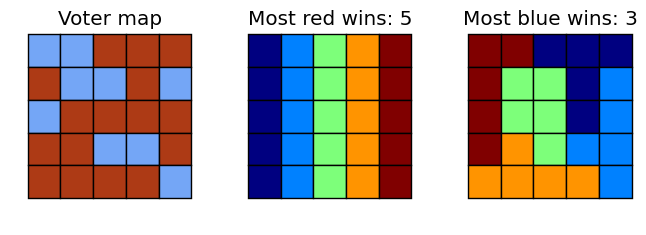## Riddler problem: rig the election... with math!¶

This was posed as a Riddler problem on Oct. 28, 2016:

Below is a rough approximation of Colorado’s voter preferences, based on county-level results from the 2012 presidential election, in a 14-by-10 grid. Colorado has seven districts, so each would have 20 voters in this model. In each district, the party with the most votes will win. The districts must be non-overlapping and contiguous (that is, each square in a district must share an edge with at least one other square in the district). What is the most districts that the Red Party could win? What about the Blue Party? (Assume ties within a district are considered wins for the party of your choice.)

The code below uses integer programming to solve the 5-by-5 example of the problem given on the Riddler website. Unfortunately, the 14-by-10 example turns out to be too complex and my code could not solve it in reasonable amount of time.

Laurent Lessard

In :
using JuMP, PyPlot, PyCall, Gurobi
@pyimport matplotlib.patches as patch
global M,N,D,C,S,W


### Board dimensions and other parameters¶

In :
M,N,D = 5,5,5             # grid size and number of districts
C = M*N                   # total number of cells
S = round(Int,C/D)        # size of each district (should be integer!)
W = floor(Int,(S+1)/2)    # votes required for a win in a district

V = [ 1 1 0 0 0
0 1 1 0 1
1 0 0 0 0
0 0 1 1 0
0 0 0 0 1 ]

v = sparse(V[:])          # vectorized version of the grid
;


### Helper functions used later in the code¶

In :
function getneighbors(p)
i,j = ind2sub((M,N),p)
neighbors = []
if i >= 2
push!(neighbors,sub2ind((M,N),i-1,j))
end
if i <= M-1
push!(neighbors,sub2ind((M,N),i+1,j))
end
if j >= 2
push!(neighbors,sub2ind((M,N),i,j-1))
end
if j <= N-1
push!(neighbors,sub2ind((M,N),i,j+1))
end
neighbors
end

A = zeros(C,C);
for p = 1:C
neighbors = getneighbors(p)
for n in neighbors
A[p,n] = 1
end
end

# create incidence matrix
E = length(find(A))   # number of edges
B = zeros(C,E)
for (e,c) in enumerate(find(A))
p,q = ind2sub((C,C),c)   # p and q are start and end cells for edge
B[p,e] = 1
B[q,e] = -1
end
B = sparse(B)

# draw a color-coded matrix of 1:S
function drawboard(mat)
cfig = figure(figsize=(N/2,M/2))
ax[:set_aspect]("equal")
ax[:axis]("off")

for i = 1:M
for j = 1:N
col = mat[i,j]==1 ? [116,166,246]/255 : [173,58,21]/255
c = patch.Rectangle([j-.5,(M-i+1)-.5],1,1,fc=col)
end
end
dx = 0.4
axis([dx,N+1-dx,dx,M+1-dx])
tight_layout()
return
end

# draw a color-coded matrix of 1:S
function drawsolution(mat,color_choice="jet")

# define colors
c = ColorMap(color_choice)
cols = c(linspace(0,1,D))

cfig = figure(figsize=(N/2,M/2))
ax[:set_aspect]("equal")
ax[:axis]("off")
for i = 1:M
for j = 1:N
col = cols[mat[i,j],1:3][:]
c = patch.Rectangle([j-.5,(M-i+1)-.5],1,1,fc=col)
end
end
dx = 0.4
axis([dx,N+1-dx,dx,M+1-dx])
tight_layout()
return
end
;

function evalboard(Vtest)
vtest = Vtest[:]
count = 0
for i = 1:D
sel = find(vtest.==i)
if length(sel) != S
println("ERROR: incorrectly sized subset")
end
if sum(v[sel]) >= W
count = count + 1
end
end
count
end

function drawthree(mat_board,sol_red,sol_blue,color_choice="jet")

dx = 0.4

cfig = figure(figsize=(3N/2,M/2))
ax[:set_aspect]("equal")
ax[:axis]("off")
for i = 1:M
for j = 1:N
col = mat_board[i,j]==1 ? [116,166,246]/255 : [173,58,21]/255
c = patch.Rectangle([j-.5,(M-i+1)-.5],1,1,fc=col)
end
end
axis([dx,N+1-dx,dx,M+1-dx])
title("Voter map")
tight_layout()

c = ColorMap(color_choice)
cols = c(linspace(0,1,D))

ax[:set_aspect]("equal")
ax[:axis]("off")
for i = 1:M
for j = 1:N
col = cols[sol_red[i,j],1:3][:]
c = patch.Rectangle([j-.5,(M-i+1)-.5],1,1,fc=col)
end
end
axis([dx,N+1-dx,dx,M+1-dx])
title(string("Most red wins: ", D-evalboard(sol_red)))
tight_layout()

ax[:set_aspect]("equal")
ax[:axis]("off")
for i = 1:M
for j = 1:N
col = cols[sol_blue[i,j],1:3][:]
c = patch.Rectangle([j-.5,(M-i+1)-.5],1,1,fc=col)
end
end
axis([dx,N+1-dx,dx,M+1-dx])
title(string("Most blue wins: ", evalboard(sol_blue)))
tight_layout()
return
end
;


### Solution using integer programming¶

In :
m = Model(solver=GurobiSolver(OutputFlag=0))

@variable(m, x[1:C,1:D], Bin)    # x[i,j] = 1 if cell i belongs to district j
@variable(m, w[1:D], Bin)        # w[j] = 1 if district j is a winner

for i = 1:C
@constraint(m, sum{x[i,j], j=1:D} == 1)                     # each cell belongs to exactly one district
end
for j = 1:D
@constraint(m, sum{x[i,j], i=1:C} == S )                    # each district contains exactly S cells
@constraint(m, sum{x[i,j]*v[i], i=1:C} ≥ W*w[j])            # if a district wins, there must be enough votes
@constraint(m, sum{x[i,j]*v[i], i=1:C} ≤ (S+1)*w[j]+(W-1))  # if there are enough votes, then the district wins
end

# add max-flow model to ensure connectedness
@variable(m, 0 <= f[1:E,1:D] ≤ S, Int )        # flow along each edge. integer variable not needed, but faster this way
@variable(m, fout[1:C,1:D], Bin)               # master out edges

Btmp = 1/(5*S+2)*abs(B)
for j = 1:D
@constraint(m, sum(fout[:,j]) == 1)                 # single outflow node
@constraint(m, B*f[:,j] + S*fout[:,j] .== x[:,j] )  # flow conservation equations
@constraint(m, x[:,j] .>= Btmp*f[:,j] )             # if edges are used, so are the associated nodes
end
;


### Optimize blue and red¶

In :
# optimize red
@objective(m, Min, sum(w))
@time solve(m)
println("Max of ", D - getobjectivevalue(m), " districts won for Red\n")
xx = round(Int,getvalue(x))
val = xx*collect(1:D)
sol_red = reshape(val,M,N)

# optimize blue
@objective(m, Max, sum(w))
@time solve(m)
println("Max of ", getobjectivevalue(m), " districts won for Blue")
xx = round(Int,getvalue(x))
val = xx*collect(1:D)
sol_blue = reshape(val,M,N)
;

  0.038953 seconds (191 allocations: 348.969 KB)
Max of 5.0 districts won for Red

0.193381 seconds (71 allocations: 77.828 KB)
Max of 3.0 districts won for Blue

In :
drawthree(V,sol_red,sol_blue)
tight_layout()
savefig("gerry_solutions_small.png")In [ ]: